+
Order shopping_cart

# Fisher Effect

Fisher effect is that which proposes that, if the real interest rate equals the nominal interest rate minus the expected inflation rate, and if the real interest rate were to be constant, then the nominal rate and the inflation rate have to be adjusted on a one-for-one basis. A simpler definition would be an increase in inflation leads to an increase in the nominal interest rate. As an example, if the real interest is maintained at a constant of 5.5 per cent and inflation then increased from 2 per cent to 3 per cent, the Fisher Effect shows that the nominal interest rate would then increase from 7.5 per cent (5.5 per cent plus 2 per cent inflation rate) to 8.5 per cent (5.5 per cent rate plus 3 per cent inflation rate).

In the same manner, the Fisher effect also refers to the theory that, the nominal interest rate (the actual monetary interest rate, which is expressed as r) in a country is determined by the real interest rate (inflation-adjusted rate, which is expressed as R) and the inflation rate i as expressed in the following formula: (1 + r) = (1 + R) (1 + i)

If the real interest rate is 5 percent, the U.S. inflation rate is at 3 percent, and the inflation rate of the euro area (the countries that use the euro) is at four percent, what are the nominal interest rates for both the United States and the euro area?

GET A PRICE QUOTE

I’m new here 15% OFF

For the United States, the nominal interest rate is

(1+ r)= (1+ 3) (1+ 5)

(1+ r) = (4) (6)

(1+r)= 24

r-1= 24-1

r-1+ 1 = 23

r=23

Interpret the calculation for your trainees.

Using the formula (1+r) = (1+R) (1+i), replace the R with 3, and I with 5

After multiplying four and six, the r will be the only remaining factor

Eliminate one by subtracting one from both sides and the answer remains 23

What are at least 3 implications of exchange rate fluctuations for Axetem as they relate to marketing and production decisions?

Exchange rate fluctuations do actually affect the economy of a country and therefore the businesses there. An appreciation of the exchange rate has both short term and long term effects on various businesses in a particular country. Some of the major beneficiaries and losers start to show themselves as an economy adjusts itself to a higher exchange rate. Firstly, a strong dollar translates to lower import prices, which will then boost the real living standards of the consumers in the short run. This is by increasing the purchasing power of the residents. Secondly, a strong dollar would translate to cheaper access to imports of raw materials, components as well as capital inputs. This is especially good for businesses that depend on imported components such as Axetem International, Inc. Thirdly, a strong exchange rate would help a lot in controlling inflation hence good environment for Axetem International, Inc.

In conclusion, the fisher effect can also be said to be in a model where inflation is supposed to be steady, the nominal interest rate changes one-for-one with the inflation rate. On the other hand, the empirical analogy will be the Fisher hypothesis. The nominal interest rate (the actual monetary interest rate, which is expressed as r) in a country is determined by the real interest rate (inflation-adjusted rate, which is expressed as R) and the inflation rate i as expressed by the following formula:

(1 + r) = (1 + R) (1 + i)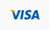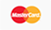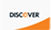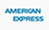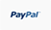Discount applied successfully